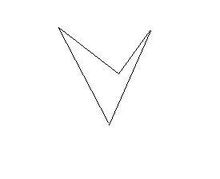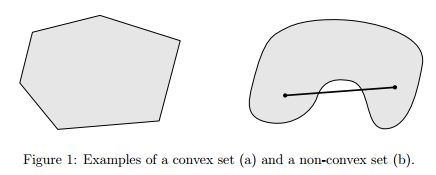# 1 高数教材中拉格朗日乘子法的泛化

## 1.1 高数教材中的拉格朗日乘子法

{minf(x)s.t.hi(x)=0i=1,2...,n

min[f(x)+i=1nλihi(x)](1)

## 1.2 KKT（Karush–Kuhn–Tucker conditions）问题

s.t.gi(x)=0i=1,2...,ps.t.hj(x)0j=1,2...,qf(x)=i=1pμigi(x)+j=1qλjhj(x)λj0j=1,2...,qλjhj(x)=0j=1,2...,q

# 2 凸函数## 2.1 仿射，凸集## 2.2 凸函数

• 凸集合交集是凸集
• 凸函数的定义是根据凸集来的：
f(x)是凸函数，则有
f(λx1+(1λ)x2)λf(x1)+(1λ)f(x2)

f称为I上的凸函数，当且仅当其上境图（在函数图像上方的点集）为一个凸集
• 凸函数与我们以前接触的函数不一样。因为是翻译问题，数学上的因为形状凹下去，所以将Convex翻译为凹函数，但Convex Set又叫凸集合来定义的。这里有必要把老版本翻译的凹函数凸过来。

## 2.3凸函数的性质

• 一元二阶可微的函数在区间上是凸的，当且仅当它的二阶导数是非负的；这可以用来判断某个函数是不是凸函数。如果它的二阶导数是正数，那么函数就是严格凸的，但反过来不成立。根据上节课的经验，当一元变为多元时，可以判断Hassion矩阵是否正定。
• 保凸运算（这个很重要）
1. f是凸函数，自变量的线性组合，f(Ax+b)也是凸函数
2. f1,,fm$f_1,\cdots,f_m$为凸函数，w1,,wm0$w_1,\cdots,w_m \ge 0$,则mi=1wifi$\sum_{i=1}^{m} w_if_i$也是凸函数
3. f1,,fm$f_1,\cdots,f_m$为凸函数，逐点最大f(x)=sup{f1(x),,fm(x)}$f(x)=sup\{f_1(x),\cdots,f_m(x)\}$也是凸函数。这条性质跟实际应用时可以给正则化一个解释。加了正这则化的凸函数也是凸函数，所以正则化对原函数的解题方法无影响。
4. g,h为凸函数，扩展的h非递减（这条性质很重要）。则f(x)=h(g(x))也是凸函数。
• 对于凸函数f的α$\alpha$水平子集Sα={x|f(x)a}$S_\alpha = \{x | f(x) \le a\}$是凸集。水平子集是凸集的函数不一定是凸函数。这样的函数称为拟凸函数。

## 2.2 凸优化问题

minimizef(x){s.t.gi(x)=0i=1,2...,ps.t.hj(x)0j=1,2...,q

1. 目标函数是凸函数我们的目标函数f(x)是凸函数
2. 不等式约束函数也是凸函数，这里要注意不等号的方向。有些数不等号方向不同，这里一定要注意。这条要求的实质条件是围成的可行域交集是个凸集。由于具有不同的形式，在后面推导KKT的过程会有不同。
3. 等式约束（这里的gj(x)$g_j(x)$）必须是仿射的。

# 3 KKT（Karush–Kuhn–Tucker conditions）问题的解释——对偶问题

## 3.1 普通的条件极值问题

注意：目标函数*“最小化问题”*与h函数的*“不等号方向”*是配对的；

minimizef(x){s.t.gi(x)=0i=1,2...,ps.t.hj(x)0j=1,2...,q

L(x,μ,λ)=f(x)+pi=1μigi(x)+qj=1λjhj(x)$L(x,\mu,\lambda)= f(x)+\sum _{i=1}^{p}\mu _{i}g_{i}(x)+\sum _{j=1}^{q}\lambda _{j}h_{j}(x)$

λ0,x0$\lambda \geqslant 0 , x_0$为一个区域内符合条件的点时，我们取区间D内x的逐点最小值，就是下确界inf，有：
g(μ,λ)=infxDL(x0,μ,λ)=f(x0)+i=1pμigi(x0)+j=1qλjhj(x0)

L(x,μ,λ)$L(x,\mu,\lambda)$是关于μ,λ$\mu,\lambda$的仿射函数（我们暂定算凹的），那么g(μ,λ)$g(\mu,\lambda)$应该算凹（或者仿射）吧，但肯定是非凸的。

g(μ,λ)f(x0)+i=1pμigi(x0)+j=1qλjhj(x0),g(μ,λ)f(x0)

g(μ,λ)f(x)=p

## 3.2 对偶问题

maximizeg(μ,λ)s.t.λ0

但是当“强对偶”现象情况发生时，等号成立。